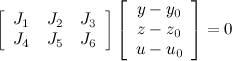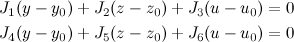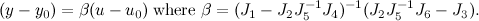# Taylor-Linearization that eliminates intermediate variables.

 0 This must seem like a really elementary question, so please bear with me. Suppose I have a nonlinear system of equations: f(x) = 0  where x = [ y z u ]'. y = vector of output variables, dimension m; z = vector of intermediate variables, dimension n; u = vector of input variables, dimension p. The dimension of f is m+n. When u is specified, the system is fully specified. Question. How do I construct a linearization about some point x0 = [ y0 z0 u0 ]' such that: y - y0 = B * (u - u0)  and there are no intermediate variables z present in the final form? Motivation. I have a huge model f(x) in my optimization problem that relates y and u in a fairly linear way, at least in the neighborhood of a point of interest x0. The vectors y and u are fairly small, but the vector z is huge. I'd like to derive a parsimonious model that does not involve z at all. I know this can be done quite easily by fitting a multilinear regression model between y and x for a set of data generated from evaluating f(x), but since I have the algebraic form for the full nonlinear model, I prefer to do a Taylor linearization instead. My progress so far. My attack is as follows: Write the Taylor-expansion for f(x) f(x0) + J(x0) * (x - x0) = 0 where J = Jacobian of f(x). For a specified u0, we can solve the system f(x) to obtain x0, our linearization point. As a result, f(x0) = 0 because x0 is a solution of the system. The above linearization equation simplifies to: J(x0) * (x - x0) = 0 In order to eliminate the intermediate variables z, we could in theory partition the Jacobian as follows:If we write this out in full, we get:Isolating and eliminating (z - z0), and rearranging, we get:Unfortunately, J5 etc. are not necessarily square, nor invertible. Is there a better way to do the partitioning? Can we apply the basic/non-basic partitioning method in GRG (Generalized Reduced Gradient) methods to this problem? How? Edit: corrected a mistake in the above equation. asked 30 Aug '10, 17:53Gilead ♦ 2.3k●5●14 accept rate: 15%

 1 J5 is not square? Don't J1, J2 and J3 have m rows and J4, J5 and J6 have n rows? Anyway, let M = [J2', J5']', which I think is (m+n) by n. Is it rank n? answered 30 Aug '10, 19:00Paul Rubin ♦♦ 14.6k●5●13 accept rate: 19% Of course! I knew the column-wise partitioning was m|n|p, but I couldn't figure what the row-wise partitioning was (in retrospect, it was staring me in the face! m|n gives a square matrix J5, and all the other inversions will work out). My bad. Thanks for pointing that out. I should mention that J5 can sometimes be rank-deficient -- but that's easily handled using a pseudoinverse. Thanks again! (30 Aug '10, 19:29) Gilead ♦
 toggle preview community wiki

By Email:

Markdown Basics

• *italic* or _italic_
• **bold** or __bold__
• image?![alt text](/path/img.jpg "Title")
• numbered list: 1. Foo 2. Bar
• to add a line break simply add two spaces to where you would like the new line to be.
• basic HTML tags are also supported

Tags: